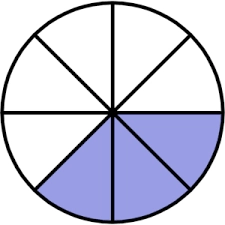# Minus fractions

Evaluate the expression shown below and write your answer as a fraction in simplest form.

minus, start fraction, 2, divided by, 11, end fraction, minus, start fraction, 11, divided by, 10, end fraction

x =  -141/110

### Step-by-step explanation:Did you find an error or inaccuracy? Feel free to write us. Thank you!

Tips for related online calculators
Need help calculating sum, simplifying, or multiplying fractions? Try our fraction calculator.